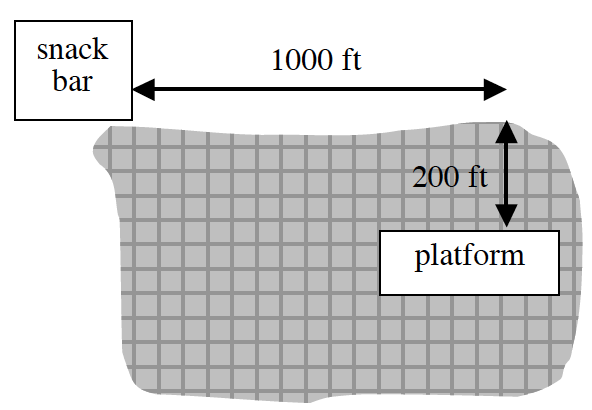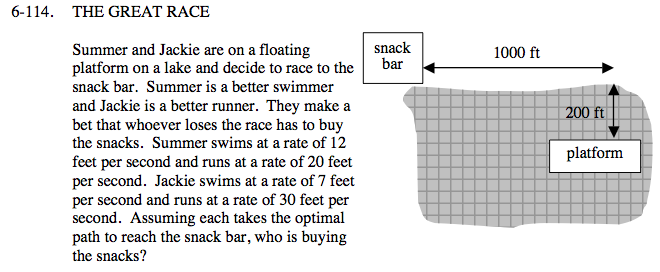### Home > CALC > Chapter 6 > Lesson 6.3.4 > Problem6-114

6-114.
1.THE GREAT RACE

2. Summer and Jackie are on a floating platform on a lake and decide to race to the snack bar. Summer is a better swimmer and Jackie is a better runner. They make a bet that whoever loses the race has to buy the snacks. Summer swims at a rate of 12 feet per second and runs at a rate of 20 feet per second. Jackie swims at a rate of 7 feet per second and runs at a rate of 30 feet per second. Assuming each takes the optimal path to reach the snack bar, who is buying the snacks? Homework Help ✎In order to optimize the time it takes to reach the Snack Bar, both girls will swim part of the way and run part of the way. Notice that the running part is horizontal but the swimming part is diagonal.

d(x) = (distance swimming) + (distance running) = (hypotenuse of triangle) + (horizontal line)

Since distance = (rate)(time),

$\text{time}=\frac{d(x)}{\text{rate}}.$

Write specific t(x) equations for each girl.

Before you apply Calculus to optimize, write a geometric equation describing the distance, d(x), of a generic journey to the snack bar. Let x represent the distance on land that each girl is NOT running.

Convert the distance equation into time equations, t(x).

Setting t'(x) = 0 will give you the optimal value of x for each girl. In other words, how far each girl should swim and run. Use that information to calculate the time it takes each girl to reach the snack bar.

Use the eTool below to explore the problem.
Click on the link to the right to view to full version of the eTool. Calc 6-114 HW eTool